# Skills in mathematics

+1 vote
2 lessons

Estimating the weight of different objects can be successfully done if we compare them to an object of the same type, or a similar type made of the same materials.

Milk is an interesting example.
Milk is a liquid and like most everyday liquids it consists almost entirely of water - 87%, the rest is protein, milk fat, lactose, micronutrients like calcium etc. So we will estimate that milk is a little bit heavier than water. One liter of water is exactly one kilogram in weight, that is the density of water -  1 kg/l. So we can say that a liter of milk has slightly the same density (weight per kilogram), maybe a little above but close.Image source: https://www.inchcalculator.com/water-weight-calculator/

If we check it out it turns out one liter of milk is 1 kilogram and 30 grams, so for smaller amounts we can even approximate the weight of milk with a weight of water.
In fact we can approximate the weight of any liquid this way as long as it has similar density as water, we can estimate the density by examining the thickness of the substance though this is not entirely correct but for an estimation it is ok.

So a two liter bottle of juice, for example will most likely weigh around 2 kilograms as juices are almost entirely water.

2 lessons

First let's see a very nice way how to practically prove that triangles always have 180° sum of angles.
Check out this video:

Cool right? You can try this yourself with any triangle you care to cut out.
Try and see if you can make one whose angles don't add up to 180.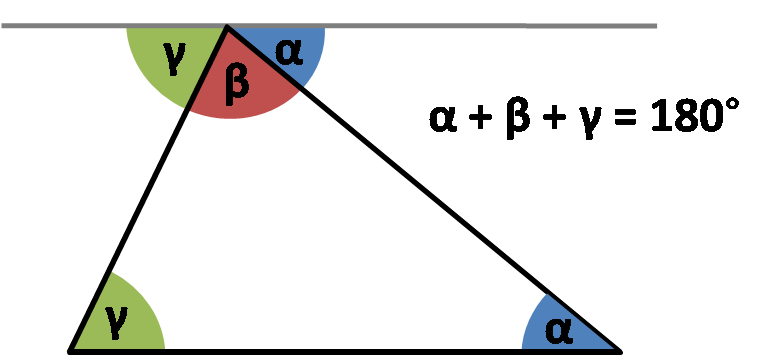Image source: Omnicalculator.com

This means that at all times we can calculate the third angle if we know the other two in any triangle.

So let's try this out!

EXERCISE:

Let's say we have a triangle just like one in the image above and let's say it's known angles are α=25°, β=75° (this doesn't necessarly have to be true for the image above, we use triangle images often more as a sketch than an actual drawing). The third angle, angle γ is unknown and we need to find it. Therefore we write:

α=25° β=75° γ=?

α + β + γ = 180°

γ = 180°- (α + β)

α + β = 25° + 75° = 100°

γ = 180°- 100°
γ = 80°

So we calculated the third angle by substracting the sum of first two from 180°.

+1 vote
1 lesson
all average values are integer numbers check out this video for a none integer average.

+1 vote
1 lesson

A perimeter of a geometric shape would be the path we would have to travel if we would walk around the shape on its edges.
Simply speaking in order to figure out a perimeter of a geometric shape we need to know the lenght of all of its edges and sum these lengths together.

Irregular geometric shapes make it difficult as we have to know each and every edge in order to make the calculation. So if have a irregular geometric shape with four diffrent egdes : a,b,c,d... The perimetire would simply be:

P = a + b + c + d

A good example of this you can find on this link

With regular geometric shapes it is a little bit easier as they follow some rules. For example if we take rectangle, it is a geometric shape that is by definition made of four egdes, out of two and two are equal.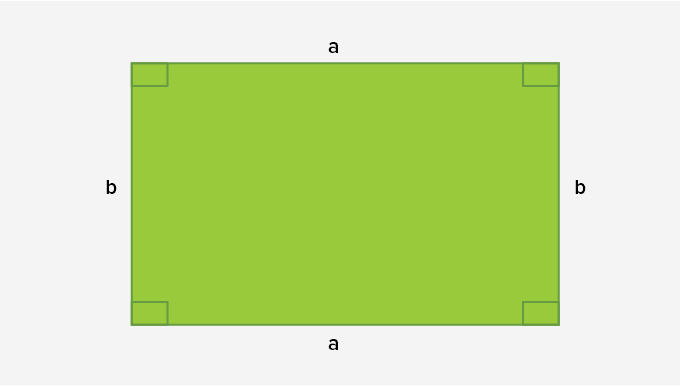Image sourced: https://www.splashlearn.com/math-vocabulary/geometry/rectangle

Taking that into account, we would write that perimeter of a rectangle is a sum of its four egdes:

P = a + a + b + b

or simpified

P = 2 x a + 2 x b

Which makes it a lot easier.

Let's try it on an examle.
Let's try and find a perimeter of some rectangles.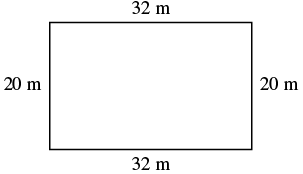Here we can se we have edge a= 32 m and edge b= 20 m (here we also use units which doesn't change things a lot).

So our perimeter should be the sum of all edges.

P = 2 x a + 2 x b
P = 2 x 32m + 2 x 20m = 64m + 40m
P = 104m

So our perimeter is 104 m and this is the procudere we will use whenever we have to calculate perimiter of a rectangle.

To find out more about calculating perimeter check out this interesting video:

1 lesson

Mellan två linjer som möter varandra finns alltid en vinkel. Vi skiljer på tre olika sorters vinklar: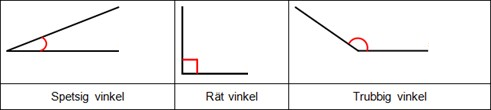Tänk på att linjernas längd inte spelar någon roll, bara hur de möter varandra. Linjerna kallas vinkelben. Den punkt de möts på kallas vinkelspets.
Vi mäter vinklar i grader. Ett helt varv är 360 grader. Ett halvt varv är 180 grader.Ett fjärdedels varv är:

Thanks to Matteboken

+1 vote
1 lesson

A video in english that teaches multiplaying with the box or grid method

And a video for the Lattice method

Thanks to Let's Do Math

2 lessons
+1 vote
1 lesson

Here is a video in english that describes how to use short division

Thanks to Let's Do Math

Here is a version in swedish using staircase- and the chair method:

Thanks to Eddler

1 lesson

## What is Fraction?

Fractions represent equal parts of a whole or a collection.

Fraction of a whole: When we divide a whole into equal parts, each part is a fraction of the whole.

For example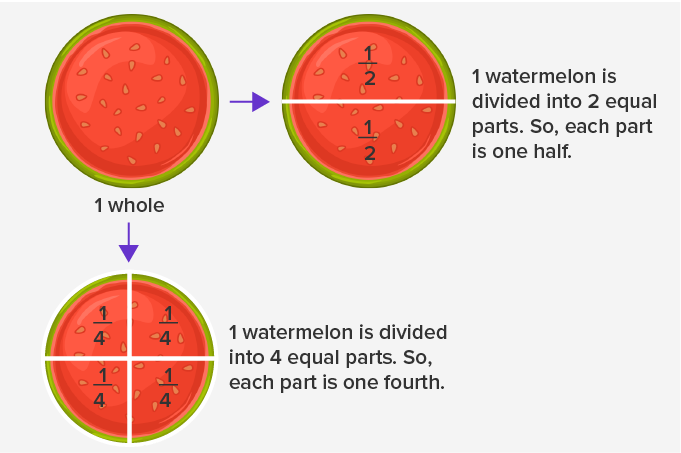Powerd by splashlearn.com
https://www.splashlearn.com/math-vocabulary/fractions/fraction

+1 vote
1 lesson

The meter is the base unit from smaller units are dm, cm and mm. The prefixes deci, centi and mili that we put before meter come from latin idicating deci (like decimal) - tenth part, centi - (like century) one hudreth part and mile (as mille in italian which means thousand) a one thousandth part.

For easy conversion have in mind that we use decimal system meaning step of conversion is ten. If we are converting to smaller units we multiply with than for each step of conversion because we need to get a larger number if we count in smaller units. Just as if we would count the money in cents rather than in dollars we would get a larger number though value would be the same.Picture taken from https://www.uzinggo.com/conversion-volume-measures/proportions-measurement/math-foundations-grade-6 where you can check out for more examples

If we convert from larger to a smaller unit we would multiply with ten for each step of conversion.
The exception is when we convert from meter to kilometer we devide with a thousand but that is only beacuse in between we actually have two units that we rarely use - dekameter and hectometar.

For example if we would convert 15 m to mm we would multiply with 10 to get the value in dm (150 dm) than again with 10 to get a value in cm (1500 cm) than once more with 10 to get a value in mm (15000). So for each step of conversion we multiplied with ten and since there were three steps in between we multiplied with one thousand effectively which is exactly how many milimiters there is in one meter so if we have 15 m there is 15 000 mm in it.

1 lesson

Problem solving strategy

When one comes across a prblem that seems like it's too hard to solve, many give up. However, when equipped with the right strategies, there's no problem in this world that is impossible. As the saying goes, an elephant is eaten one little piece at a time and the same goes for probolem solving. Have a look at the steps below that are meant for solving typical school math written problems.

2. What is the QUESTION? Highlight it.
3. What is known? Circle all pieces of information there are.
4. Make a drawing of the problem.
5. How can you solve the problem?
6. Write an expression
7. Calculate

Exercices:

A 30m long rope is used to make a rectangle that is 4m wider than its length. Calculate the width and length of the rectangle using a linear equation.

CDs were sold for a discount of 5,40€. Jane calculated that 7 CDs now cost the same as 5 CDs with the normal price. What is the price before the discount?

Jane, Rita and Howard get 51€ for plowing all the snow of their neighbours driveways. They split the money as follows: Rita gets 7€ more than Howard and Jane, who worked the most, gets twice the amount of Rita and Howard combined. How much money do they each get?

2 lessons

Graphing equations

Math is often ambiguous at best, especially when things get more and more complicated. However, this is also true for real life: How do you make sense of team or player statistics in sports, for example? How do the meteorologists figure out where the weather patterns are taking us? The common denominator is graphing. With visualisations we can make complicated things make sense much easier.

Let’s learn the theory:

Exercises:

1. Look at the graph. What is…
• The largest value of the function?
• Smallest value of the function?
• Which value of x gives the the largest value?
• What is the value of the finction when x = -3?2. Graph the following equations using intercepts.

• Graph 2x + 4 = 0
• Graph 2y - 6 = 0

3. Graph the following three equations on the same set of coordinate axes.

• y=x+1y=2x+1y=−x+1

4. Graph the following three equations on the same set of coordinate axes.

• y=2x+1y=2xy=2x−1

Credit: math.libretexts.org

2 lessons

Statistics: Mean, median and mode

When looking at box scores of your favourite NHL, NBA, Champions League or F1 racing team, it is important to understand what kind of descriptors are used to make sense of the big set of numbers that are collected. For example, it might be important to know how many times a point guard loses the ball in a basketball match or how fast an F1 driver drives around a certain track. But how can we compare different point guards or F1 drivers to know which one is best and gets the contract or a place in the starting five. This is when mean, median and mode come in handy.

Let’s learn the theory:

Exercises:

1. Scores in a math test were 16, 22, 19, 22, 20, 15, 18, 17 and 24.

• Calculate the mode
• Calculate the median
• Calculate the mean

2. Grade in all the math test throughout the academic year for John were 8-, 8+, 7½, 8+, 7, 8½ and 9

• Calculate the mode
• Calculate the median
• Calculate the mean

2 lessons

Geometry

Practical excercices:

1. Go around your home and take pictures of things that represent the following (Google the names first if needed!):

• Cone
• Pyramid
• Rectangular prism
• Sphere
• Tetrahedron
• Cylinder
• Cuboid

2. Draw a floor plan of your dream house. Use at least the following shapes in your plan and name them in your drawing.

• Circle
• Square
• Rectangle
• Parallelogram
• Trapezoid

3. Practice using the compass by drawing these beautiful mandala shapes.

Let’s learn the theory:

Exercises:

1. Draw a circle and mark the following parts in it:

• Diameter
• Chord
• Circumference

2. Try to find as many objects around you that are shaped like a circle. Take some thread and measure their circumference and diameter. Plot the results in a graph (example below). What do you notice?

 Object Circum-ference Diameter Circum-ference/Diameter

3. Calculate the circumference of a circle when the radius is:

• 5,6 cm
• 12,6 m
• 1,0 km
• 0 m

4. The circumference of a circle is 74,2 m. Calculate:

• The diameter

2 lessons

Linear equation

Quite often in life, we know some variables, but we must figure out an unknown. For example, we may want to save money for a moped, but to know how long it is going to take, we must be able to calculate it according to our weekly allowance, price of the moped and perhaps other things we are going to end up buying with the money.

Practical example:

How long would it take for you to come up with the money if your weekly allowance was 10€, the price of the moped 1550€ and you would buy sweets with 2€ every week? What kind of calculations do you need to make to get the answer?

Equations are a way of writing expressions with unknown variables that can be figured out using math.

Let’s learn the theory:

Excercises:

1. Figure out the X

• x/9 = 1/3
• 20/x = 5/1
• x/12 = 2/3
• 6/16 = 3/x
• x/4 = 5/20
• 3/8 = x/24

2. A runner’s step is such that on a 100m distance he takes 83 of them. How many steps must he take to run 1,7km? Come up with the equation and solve the X.

3. Solve the equation by dividing both sides with the same number.

• 3x = 60
• 10x = -30
• -2x = 24
• -5x = -30

4. Solve the equation by multiplying both sides with the same number.

• x/3 = 4
• x/3 = -7
• x/-9 = 4

5. Solve the equation.

• x/3 = 500
• x + 9 = 13
• 12x = 11x + 3

2 lessons

Functions

Have you ever come across the problem of understanding how warm or cold it is when the temperature is given in Fahrenheit or vice versa, if you live in the US, in Celsius? Wouldn’t it be convenient if you could have a machine that would do the conversion for you? This is what functions do: You input something, and it is given as output as something else according to a rule.

Let’s learn the theory:

Excercises:

1. A function subtracts 9 from a number. What is the output of the function when input is 15? 45? 9? 5?

2. Calculate ƒ(x) = 5x + 1 when x = 7
x = 10
x = -1
x = -7

3. Calculate ƒ(x) = -3x + 10 when
x = 2
x = 12
x = 0
x = -6

3. For ƒ(x) = x – 6, which value of x gives the output of
5
0
-4
-12

+1 vote
3 lessons
+1 vote
2 lessons
2 lessons

How to calculate to get the probability of getting 11 with two dice

Thanks to Don't Memorise

+1 vote
2 lessons

Number Sequences

Patterns are everywhere in the world. Maybe the most famous pattern that appears everywhere in the world from nature to fine arts is the Fibonacci sequence. Have a look at the following video to learn more. What kind of repeating patterns can you find at home?

Let’s learn the theory:

Exercises:

1. Continue the string of letters two letters so that the string makes sense.

• A, D, G, J, ___, ___
• V, R, N, J, ___, ___
• A, E, I, O, U, ___, ___

2. How many sticks will there be in the fourth figure?3. Continue the string of numbers with 3 more numbers.

• 17, 15, 11, …
• 56, 53, 50, 47, …
• 10, 7, 4, 1, …

4. Write the first for numbers of the sequence according to the following rules:

• First number is 2 and the following number is always 7 bigger than the previous one
• First number is 1 and the following numbers are always three times bigger than the previous
• 3 – the place of the number in the sequence + 40

5. What is the missing number?

• 2, 3, ---, 5, 6, …
• 5, 2, -1, ___, -7…
• 500, ___, 20, 4, …

+1 vote
1 lesson

Linear differential equations

Let’s learn the theory:

Excercises:

math.libretexts.org Differential Equations

+1 vote
1 lesson

Interest and amortization

Once you enter adulthood, you will be faced with many responsibilities. You will have to understand new concepts that have a direct effect on your life. Understanding finances can save you a lot of money and help you make wise decisions. Buying your first house or apartment might happen sooner than you think. It’s worth getting ready to understand how to calculate interest and amortization.

Let’s learn the theory:

Exerecises:

1. Chris Columbus bought a house for $293,000. He put 20% down and obtained a simple interest amortized loan for the balance at % 8 3 5 annually interest for 30 years. 2. Find the amount of Chris’s monthly payment. 3. Find the total interest paid by Chris. 4. Most lenders will approve a home loan only if the total of all the borrower’s monthly payments, including the home loan payment, is no more than 38% of the borrower’s monthly income. How much must Chris make in order to qualify for the loan? 5. Complete an amortization table for the first 2 months of the loan, the 180th through 181th months (only find the balance due for the 180th month) of the loan, and the final 2 months (only find the balance due for the 359th month) of the loan. https://www.asu.edu/courses/mat142ej/finance/examples/Amortized_Loan_Example.pdf Find the answers and more examples here. +1 vote 3 lessons +1 vote 1 lesson Rate of change Everything that you study in Math has a practical application. Here are some practical applications for average rate of change: • Rocket science and space travel • Chemical Engineering Calculations • Predicting your electricity bill: If you have an idea of the average rate in which electricity is consumed in your household, then you can predict your electricity bill for a month. Let’s learn the theory: Here’s a good link that explains the topic with clear examples: +2 votes 1 lesson Probability Understanding probability is a useful skills. It allows you to make informed choises that are not based on subjective feelings but an understanding of reality. Let's revise the theory: Here's an example of a real life situation where probability is useful. ## Sports Strategies Athletes and coaches use probability to determine the best sports strategies for games and competitions. A baseball coach evaluates a player's batting average when placing him in the lineup. For example, a player with a 200 batting average means he's gotten a base hit two out of every 10 at bats. A player with a 400 batting average is even more likely to get a hit – four base hits out of every 10 at bats. Or, if a high-school football kicker makes nine out of 15 field goal attempts from over 40 yards during the season, he has a 60 percent chance of scoring on his next field goal attempt from that distance. The equation is: 9/15 = 0.60 or 60% Source +3 votes 1 lesson Calculating average speed and distance travelled Mathematical equations can be used to solve many everyday life situations. Calculating the average speed of an object is one of them. However, perhaps a much more useful skill is the ability to tell someone who is waiting for you to finish your journey, at what time you will arrive. Let’s learn the theory: Here’s a great website with example problems and instructional videos +1 vote 1 lesson +1 vote 1 lesson Rotational Symmetry Let’s learn the theory: Exercises: 2. What are the coordinates when the following points are mirrored according to the x-axis? • (4, 1) • (0, -5) • (-6, 0) • (-4, -7) 3. What are the coordinates when the above points are mirrored according to the y-axis? 4. Draw a triangle A(-1, 3), B(-2, 0) and C(2, -1). Mirror the triangle by the point (0, 1), What are the vertices of the mirrored triangle? +1 vote 1 lesson Prime numbers Prime numbers are numbers that are divisible only by themselves and 1. Let’s learn the theory Exercises: Here are online worksheets about prime numbers Mathscore Prime%20Numbers Mount Royal University->Higher Arithmetic->Prime_numbers +3 votes 1 lesson Pythagorean Theorem Some of the practical applications of the Pythagorean theorem include marine navigation, building almost anything (houses, bridges etc.) and figuring out which TV-size to choose (sizes are given as diagonals, so you don’t know the hight and width). Let’s learn the theory: Exercises Here are some excellent online exercises of the Pythagorean theorem: https://www.shmoop.com/squares-square-roots/pythagorean-theorem-exercises.html +2 votes 1 lesson ##Source Let’s learn the theory: Exercises: 1. A dining-table is marked for$7500 and is sold for $6000. • Find the discount given. • Find the discount given as percent. 2. In Las Vegas, Nevada, stores charge a 4.6% state sales tax and a 3.65% county sales tax. Yuki is purchasing a handbag priced at$220before tax. How much sales tax does Yuki pay for her handbag purchase?

To see more, click for the full list of skills or popular subjects.